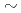Reliability assessment for the High Energy Particle Induced Radioactivity Calculation Code DCHAIN-SP 2001 was carried out through analysis of integral activation experiments with 14-MeV neutrons. The following three series of experiments conducted at the D-T neutron source facility, FNS, in JAERI were employed: (1) the decay gamma-ray measurement experiment for fusion reactor materials, (2) the decay heat measurement experiment for 32 fusion reactor materials, and (3) the integral activation experiment on mercury.As a result, it was found that the calculations with DCHAIN-SP 2001 predicted the experimental data for (1)(3) approximately within 30%, 20% and 20%, respectively. It was concluded that the activation cross section data below 20 MeV and the associated decay data as well as the calculation algorithm for solving the Beteman equation that was the master equation of DCHAIN-SP were adequate.